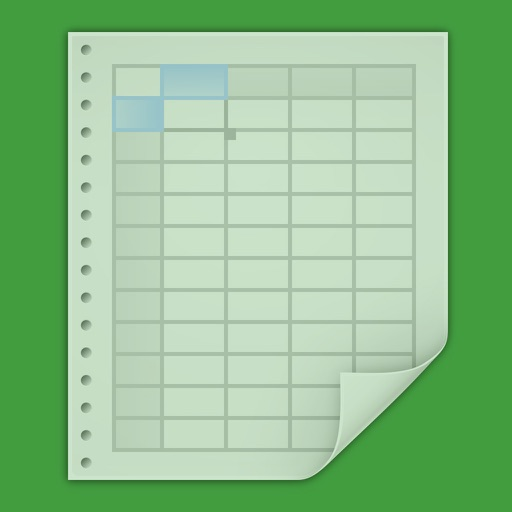## These 161 video tutorials on how to use Microsoft Excel will teach you all you need to know about spreadsheets to get yourself well up and runningby Peter Walsh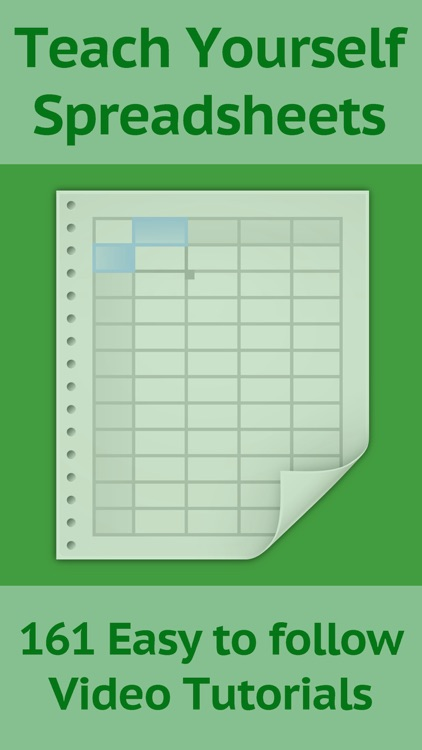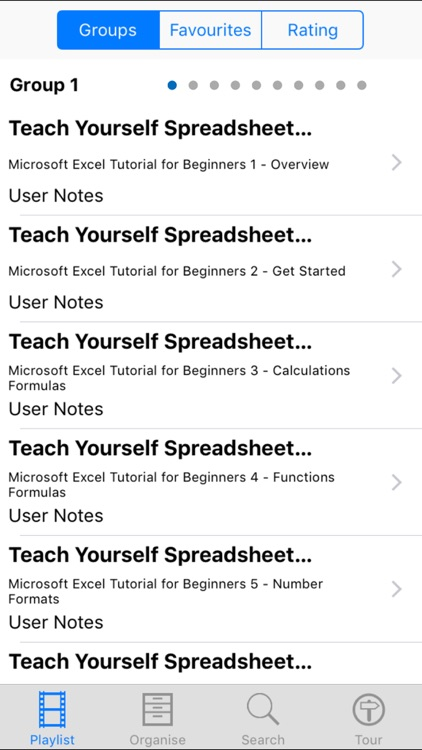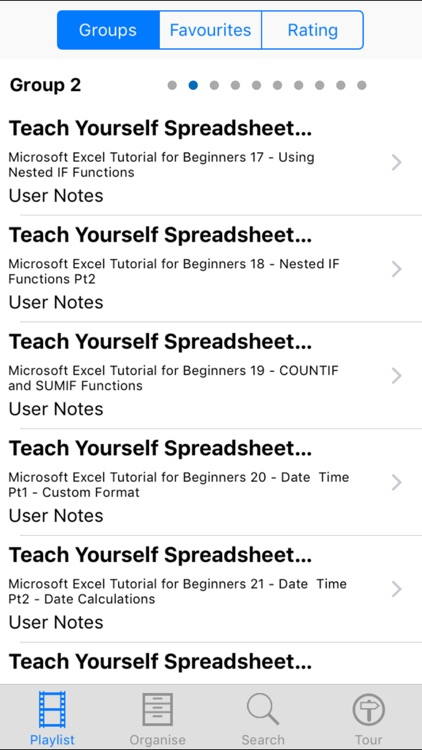These 161 video tutorials on how to use Microsoft Excel will teach you all you need to know about spreadsheets to get yourself well up and running. The lessons are aimed at beginners and cover all the very basics. It will not take you long to get a good grip on how to use a spreadsheet to make your mathmatical life easier!### App Details

Version
1.0
Rating
NA
Size
6Mb
Genre
Education Reference
Last updated
December 21, 2015
Release date
December 21, 2015

### App Screenshots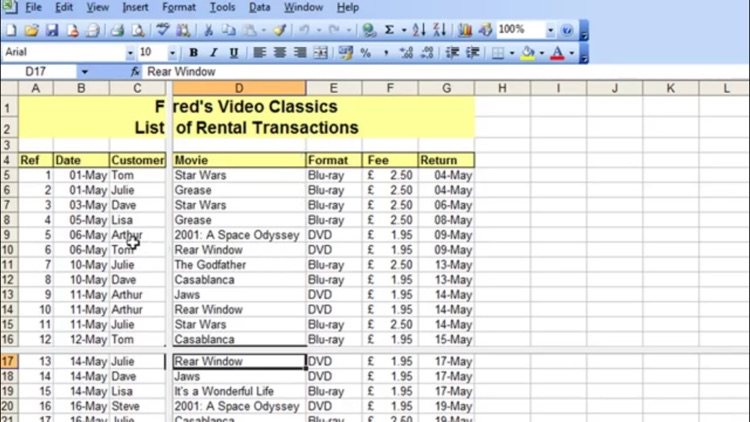### App Store Description

These 161 video tutorials on how to use Microsoft Excel will teach you all you need to know about spreadsheets to get yourself well up and running. The lessons are aimed at beginners and cover all the very basics. It will not take you long to get a good grip on how to use a spreadsheet to make your mathmatical life easier!

Lessons include
Microsoft Excel Tutorial for Beginners 1 - Overview
Microsoft Excel Tutorial for Beginners 2 - Get Started
Microsoft Excel Tutorial for Beginners 3 - Calculations Formulas
Microsoft Excel Tutorial for Beginners 4 - Functions Formulas
Microsoft Excel Tutorial for Beginners 5 - Number Formats
Microsoft Excel Tutorial for Beginners 6 - Formatting Pt1
Microsoft Excel Tutorial for Beginners 7 - Formatting Pt2
Microsoft Excel Tutorial for Beginners 8 - Formatting Pt3
Microsoft Excel Tutorial for Beginners 9 - Charts Pt1
Microsoft Excel Tutorial for Beginners 10 - Charts Pt2
Microsoft Excel Tutorial for Beginners 11 - Charts Pt3
Microsoft Excel Tutorial for Beginners 12 - Printing
Microsoft Excel Tutorial for Beginners 13 - Updating Calculations
Microsoft Excel Tutorial for Beginners 14 - Percentages and Absolute References
Microsoft Excel Tutorial for Beginners 14 Pt2 - Conditional Format Update Percentages
Microsoft Excel Tutorial for Beginners 15 - Percentages - More Examples
Microsoft Excel Tutorial for Beginners 16 - Using The IF Function
Microsoft Excel Tutorial for Beginners 17 - Using Nested IF Functions
Microsoft Excel Tutorial for Beginners 18 - Nested IF Functions Pt2
Microsoft Excel Tutorial for Beginners 19 - COUNTIF and SUMIF Functions
Microsoft Excel Tutorial for Beginners 20 - Date Time Pt1 - Custom Format
Microsoft Excel Tutorial for Beginners 21 - Date Time Pt2 - Date Calculations
Microsoft Excel Tutorial for Beginners 22 - Date Time Pt3 - IF Conditional Format
Microsoft Excel Tutorial for Beginners 23 - Date Time Pt4 - Time Calculations Formatting
Microsoft Excel Tutorial for Beginners 24 - Date Time Pt5 - Time Across Midnight Multiple Days
Microsoft Excel Tutorial for Beginners 25 - Database Pt1 - Create Lists
Microsoft Excel Tutorial for Beginners 26 - Database Pt2 - Freeze Split Table Navigation
Microsoft Excel Tutorial for Beginners 27 - Database Pt3 - Sorting Data
Microsoft Excel Tutorial for Beginners 28 - Database Pt4 - Filter with AutoFilter
Microsoft Excel Tutorial for Beginners 30 - Show Formulas on a Spreadsheet
Microsoft Excel Tutorial for Beginners 31 - Worksheets Pt1 - Multiple Worksheets
Microsoft Excel Tutorial for Beginners 32 - Worksheets Pt2 - Calculations
Microsoft Excel Tutorial for Beginners 33 - Worksheets Pt3 Sum Across Worksheets
Microsoft Excel Tutorial for Beginners 34 - Multiple Workbooks Pt1 - Calculations
Microsoft Excel Tutorial for Beginners 35 - Multiple Workbooks Pt2 - Updating Links
Excel 2013 Tutorial - Basic Graph
Excel 2013 Tutorial - Copy Cut Paste paste without formatting
Excel 2013 Tutorial - Order of Evaluation Precedence
Excel 2013 Tutorial - Introduction to Formulas and Inserting and Deleting Rows and Columns
Basic Tasks in Excel 2013 - Part 1
Basic Tasks in Excel 2013 - Part 2
Excel 2013 Tutorial - Basic Formatting Part 1
Excel 2013 Tutorial - Basic Formatting Part 2
Excel 2013 Tutorial - Number Formatting
Excel 2013 Tutorial - Personalizing the Environment Using the Backstage
Excel 2013 Tutorial - Force Printing on One Page and Navigating the Worksheet
Excel 2013 Tutorial - Auto Fill linear and growth
Excel 2013 Tutorial - More Practice with Basic Formulas and format currency
Excel 2013 Tutorial - Referencing Worksheets in Formulas and change Tab Color
Excel 2013 Tutorial - Mail Merge Ticket Numbers
Excel 2013 Tutorial - Basic Excel Word Mail Merge
Excel 2013 Tutorial Protecting Cells and Worksheets